```

```
The Tetragonal Crystal System (Part Seven)
```

```
back to Part One
```

```

## The Tetragonal-disphenoidic Class (= Sphenoidic Tetartohedric)   4*

When two hemihedrics are applied to a holohedric Form we get a so called tetartohedric Form.
This is the case with the Forms of this Class.
We obtain these Forms when we subject each holohedric Form to first a sphenoidic hemihedric (= suppression of the equatorial mirror plane and of the two vertical mirror planes that go through the horizontal crystallographic axes) and then subject the result to trapezohedric hemihedric (= suppression of all mirror planes).

The symmetry content of this Class is :

• One vertical 4-fold roto-inversion axis (4*).

### Derivation of the Forms of the Tetragonal-disphenoidic Class

MEROHEDRIC APPROACH.
The Forms of this Class will be found when we subject the holohedric Forms to the two mentioned hemihedrics.
Recall that the holohedric Forms were the following :

Protopyramid
Deuteropyramid
Ditetragonal Bipyramid
Protoprism
Deuteroprism
Ditetragonal Prism
Basic Pinacoid

Applying the sphenoidic hemihedric to these Forms resulted in the following Forms (See Part Two) :

The holohedric Protopyramid gave the sphenoidic hemihedric sphenoid (also called disphenoid).

The holohedric Deuteropyramid gave the sphenoidic hemihedric type II tetragonal bipyramid.

The holohedric Ditetragonal Pyramid gave the sphenoidic hemihedric tetragonal scalenohedron.

The holohedric Protoprism gave the sphenoidic hemihedric type I tetragonal prism.

The holohedric Deuteroprism gave the sphenoidic hemihedric type II tetragonal prism.

The holohedric Ditetragonal Prism gave the sphenoidic hemihedric ditetragonal prism.

The holohedric Basic Pinacoid gave the sphenoidic hemihedric basic pinacoid.

These sphenoidic Forms will now be subjected to the trapezohedric hemihedric. The resulting Forms are then the Forms of this Class (The Tetragonal-disphenoidic Class).

The sphenoidic sphenoid (disphenoid) will then loose its remaining mirror planes, namely the ones that bissect the angles between the horizontal crystallographic axes. The result is again a type I sphenoid because the mirror planes to be suppressed are perpendicular to the faces. See Figure 1.Figure 1. From the sphenoidic Type I Sphenoid is derived a Tetartohedric Type I Sphenoid.

The sphenoidic hemihedric type II tetragonal bipyramid does change its shape when the second hemihedric (trapezohedric hemihedric) is applied to it. It becomes a type II tetragonal sphenoid (a deuterosphenoid) See Figure 2.Figure 2. Applying the trapezohedric hemihedric to the sphenoidic hemihedric Type II Tetragonal Bipyramid does lead to a change in external shape. Its vertical mirror planes -- one is indicated by A B C -- disappear, as indicated by the coloring of the right image, and causes the change in shape, as shown in the next Figure.

The actual construction of the resulting type II sphenoid (from the right image of Figure 2) is given in the Figures 2a and 2b.Figure 2a. Construction of a Type II Sphenoid (Deuterosphenoid) from the Type II Tetragonal Bipyramid, by suppressing the yellow faces and extending the brown faces till they meet.Figure 2b. The resulting Sphenoid (the other Sphenoid can be derived from the yellow faces of the tetragonal bipyramid).

The sphenoidic hemihedric tetragonal scalenohedron yields a tritosphenoid (= type III sphenoid) in virtue of loosing its mirror planes. See Figure 3.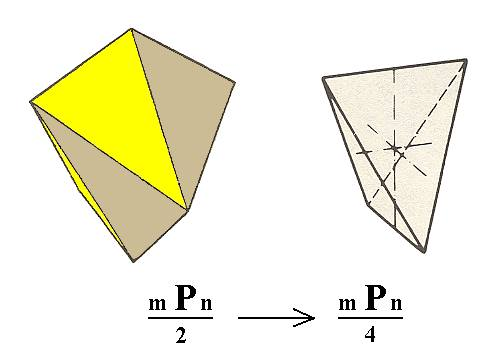Figure 3. The Tetragonal Scalenohedron becomes a Tritosphenoid when it is subjected to trapezohedric hemihedric.

The sphenoidic hemihedric type I tetragonal prism, gives, when subjected to the trapezohedric hemihedric, a tetartohedric type I tetragonal prism. See Figure 4.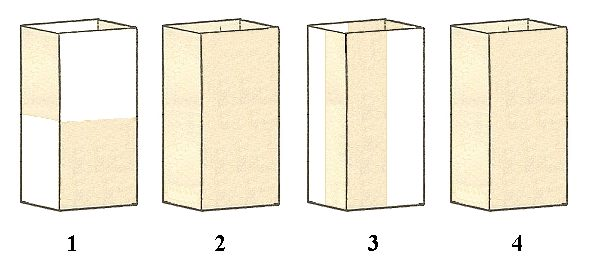Figure 4. The white 'faces' of the developing sphenoidic Protoprism (1) are suppressed while its brown faces extend till they meet, recovering the original faces of the protoprism (2), but resulting in a prism with the symmetry indicated in 1, the sphenoidic Type I Tetragonal Prism. Next (in applying the trapezohedric hemihedric to it) the remaining vertical mirror planes are removed (3), and, because of that, the white 'faces' are removed while the brown faces are allowed to extend themselves till they meet, resulting in a tetartohedric Type I Tetragonal Prism (4). The same prism is obtained when the brown 'faces' are removed while the white 'faces' are allowed to extend themselves till they meet.

The sphenoidic hemihedric type II tetragonal prism does not change its external shape when subjected to the trapezohedric hemihedric. We obtain the tetartohedric type II tetragonal prism. See Figure 5.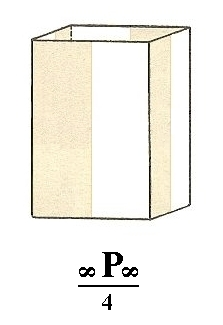Figure 5. The Deuteroprism-undergone-sphenoidic-hemihedric is subjected to trapezohedric hemihedric which means that it looses its vertical mirror planes lying between the axial planes (i.e. the vertical planes each of which containing a horizontal crystallographic axis). We imagine the white 'faces' to disappear while the brown faces extend themselves till they meet, recovering the tetragonal prism but one with lower symmetry, the Tetartohedric Type II Prism.

The sphenoidic hemihedric ditetragonal prism becomes a tritoprism ( = type III tetragonal prism). See Figure 6.Figure 6. The sphenoidic hemihedric Ditetragonal Prism, looses the mirror planes that go between the horizontal crystallographic axes. Two possible tetartohedral tetragonal Tritoprisms result, one from the (four) red faces, and one from the (four) grey faces.

The sphenoidic hemihedric basic pinacoid remains a basic pinacoid. See Figure 7.Figure 7. Derivation of the tetartohedric Basic Pinacoid from the sphenoidic hemihedric Basic Pinacoid. By suppressing the 'faces' of one color and letting extend the 'faces' of the other color, in the right image, we get this Pinacoid. It consists of two parallel horizontal faces. As the figure shows these faces are not mirror reflections of each other.

This concludes our derivation of all the Forms of the Tetragonal-disphenoidic Crystal Class by the merohedric approach.

These Forms can engage in combinations with each other, and thus it is possible that several such Forms appear in one and the same crystal.

```
```
FACIAL APPROACH

We will now derive those same Forms by subjecting the basic faces (compatible with the Tetragonal Crystal System) one by one to the symmetry operations of the present Class (the Tetragonal-disphenoidic Crystal Class).
Recall that the basic faces were the following :

•     a : a : c
•     a : ~a : c
•     a : na : mc
•     a : a : ~c
•     a : ~a : ~c
•     a : na : ~c
•     ~a : ~a : c
The only symmetry element of this Class is the 4-fold roto-inversion axis (4*).
Because the Class does not possess any mirror plane, nor any 2-fold rotation axis, the motifs, which figure in the stereographic projections, are themselves NOT symmetrical, i.e. each motif does not itself possess any mirror plane. We could represent such a motif as a comma :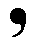, but in images of stereographic projections one normally does not so. The motifs are represented there by dots, small circles, plus signs etc. where the shape of such symbols is of no relevance. The motifs in our case are (possible crystal) faces, and they are not each for themselves symmetrical, which is detectable by means of the shape and orientation of etch pits on their surface, or by means of other physical features.
When indeed the motifs (faces) are not themselves symmetrical, the actions of the 4-fold roto-inversion axis (4*) do not imply any other symmetries, thus no mirror planes or 2-fold rotation axes appear. As the only available symmetry element in this Class the 4-fold roto-inversion axis can be depicted as in the next Figure, in which the asymmetry of the motifs is indicated.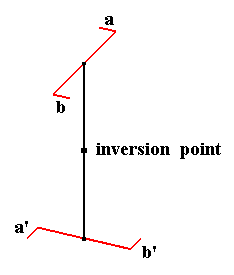Figure 8. The four-fold roto-inversion axis as the only symmetry element of the Tetragonal-disphenoidic Crystal Class.

The action of this axis is rotation by 900 about its axis followed by inversion through a point on that axis, but recall that its action can also (and totally equivalently) be described by a rotation of 900 about its axis followed by a reflection with respect to a plane perpendicular to that axis.

The stereogram of the symmetry elements of the present Class is :Figure 9. Stereographic projection of the symmetry elements and of all the faces of the general Form. Red dots upper faces, small red circles lower faces. The two straight dashed lines do not represent symmetry elements but represent the two horizontal crystallographic axes.

The face a : a : c is the unit face of the Tetragonal Crystal System. When we subject this face to the actions of the 4-fold roto-inversion axis (4*) a type I sphenoid (also called a disphenoid) (Figure 1, right image) is finally generated. We develop its stereographic projection in four consecutive steps :   We start with (the position of) the face a : a : c, then we subject it to the action of the 4* axis, which means that we rotate it 900 clockwise about the main crystallographic axis and then invert it through the origin of the system of crystallographic axes. On the result we apply again the action of that axis, and on the lastly obtained result we apply the action of that axis again. We then have generated the stereogram of the Form (generated by constructing from the initial face a configuration of faces that complies with the symmetry elements of the present Class which means that the configuration (as a whole) complies with its having a 4* axis, and not any other symmetry element whatsoever).
This same stepwise procedure we will also deploy in the generation of the stereogram of all the other Forms of this Class.Figure 10. Developing the stereogram of the Tetartohedric Type I Tetragonal Sphenoid from the face a : a : c.
Red dots are upper faces, small red circles are lower faces. The dashed straight lines do not represent symmetry elements. The horizontal and vertical (as seen in the Figure) dashed lines represent the two horizontal crystallographic axes, while the diagonal ones (as seen in the Figure) are just visual aids.

The face a : ~a : c behaves as follows under the operation of the 4* axis, generating a tetartohedric deuterosphenoid (= tetartohedric type II sphenoid) :Figure 11. Developing the stereogram of the Tetartohedric Type II Tetragonal Sphenoid from the face a : ~a : c.

The face a : na : mc yields a tritosphenoid (= type III tetragonal sphenoid) :Figure 12. Developing the stereogram of the tetartohedric Tetragonal Tritosphenoid from the face a : na : mc.

The face a : a : ~c yields a tetartohedric type I tetragonal prism :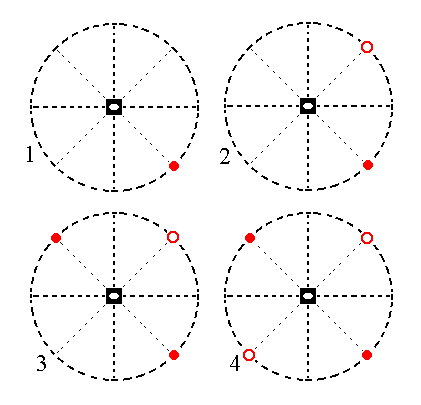Figure 13. Developing the stereogram of the tetartohedric Type I Tetragonal Prism from the face a : a : ~c.

Because the faces are vertical there is no difference between an upper face (red dot) and a lower face (small red circle). So we can symbolize the faces of the prism just as red dots, like the next Figure shows.Figure 14. Stereogram of the tetartohedric Type I Tetragonal Prism.

The face a : ~a : ~c yields a tetartohedric type II tetragonal prism :Figure 15. Developing the stereogram of the tetartohedric Type II Tetragonal Prism from the face a : ~a : ~c .
Also in this case there is no difference between upper and lower faces. So the final stereogram is as is depicted in Figure 16.Figure 16. Stereogram of the Tetartohedric Type II Tetragonal Prism.

The face a : na : ~c yields the tetartohedric tetragonal tritoprism (= type III prism) :Figure 17. Developing the tetartohedric Tetragonal Tritoprism from the face a : na : ~c. Also in this case there is no difference between upper and lower faces, so the final stereogram of this Form is as is depicted in Figure 18.Figure 18. Stereogram of the tetartohedric Tetragonal Tritoprism (= type III tetragonal prism).

Finally the face ~a : ~a : c yields again a basic pinacoid, namely a tetartohedric basic pinacoid :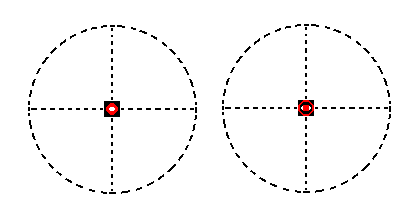Figure 19. Developing the tetartohedric Basic Pinacoid from the face ~a : ~a : c. Rotation of this face about the main crystallographic axis has no effect, but its consecutive inversion through the origin of the system of crystallographic axes does produce a second face parallel to the initial one. So the result is an upper face and a lower face which coincide on the projection plane of the stereographic projection.

```
```
This concludes the derivation of all the Forms of the Tetragonal-disphenoidic Crystal Class, and also concludes our exposition of the Tetragonal Crystal System.